# Radix Sort : Explained

### Introduction

Radix sort is a non-comparison-based stable algorithm that uses counting sort with a little modification to sort the numbers in an array.

### Why use radix sort?

We can use counting sort only when k is linear to n. It would take O(n2) time when k increases to n2 which is worse than other comparison-based sorting algorithms like merge sort, etc.

This is one of the drawbacks of counting sort and the chance for radix sort to ease the process. Radix sort can sort elements for a larger value of k in linear time.

### Properties of Radix sort

1. Linear Time Algorithm
2. Stable Sorting Algorithm
3. Non-comparison based Algorithm
4. Works for a bigger range unlike count sort.
5. Sorts elements on the basis of their digits.

Let’s see how the radix sort works.

### Approach

In radix sort, we sort numbers according to the digits i.e. from LSD [Least Significant Digit] to MSD [Most Significant Digit]. We sort the numbers according to the digits using counting sort which helps us maintain the stability of the algorithm.

Following steps are used under radix sort

1. We find the maximum number in the array i.e. the number with maximum digits.
2. The digits of the maximum number become our passes. For each pass, we run the counting sort algorithm.
3. We modify our counting sort algorithm to sort the numbers according to the digits.

### Modification of counting sort

Following modifications are done in the counting sort algorithm to make it work accordingly.

1. Declare a count array of size b, where b denotes the base of the numbers in the array.
2. Instead of directly using array elements as index in our count array, we use the digits as index in our count array.
3. Other steps remain the same as a general purpose counting sort algorithm.

Let’s see the working of this algorithm by using a simple example.

` arr[]={326,311,3,4,120,65},  n=6`

According to step 1, we find the maximum element in the array which is 326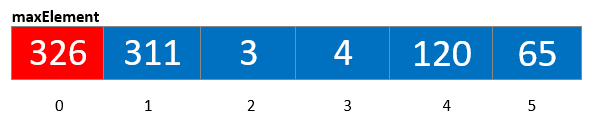According to step 2, the number of digits in the maxElement becomes the number of passes. Hence passes=3

Now for each pass, we call the counting sort function.

Under modification of counting sort, according to step 1, we declare a count array of size b, where b is the base of the elements in the array. Here b=10.

Now we use digits of our elements as the index for count array and store numbers that are smaller or equal to that digit.

For pass 1, the digits are 6, 1, 3, 4, 0, 5.

According to the above data, our count array would be as follows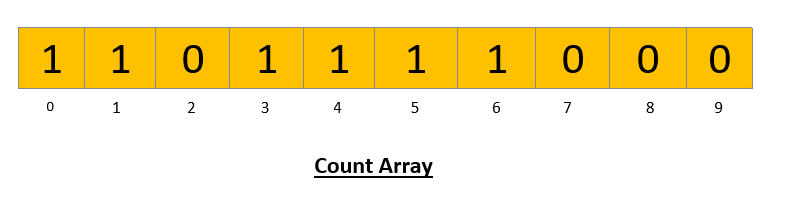Now according to the counting sort algorithm, we modify our count array. For every index/digit we find the cumulative sum of the digits smaller or equal to that index/digit. Doing this our new count array would be as follows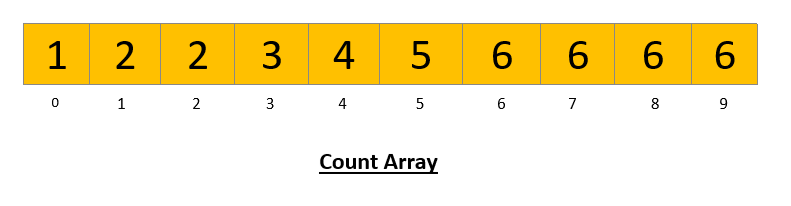This count array would help us in placing the element according to the digit.

It’s time to build our output array

Now we perform backward iteration in our array and we place our element in the output array according to its last digit using the modified count array.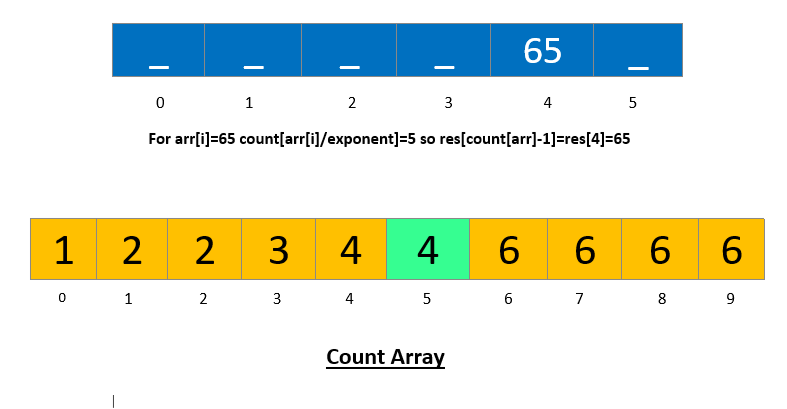Doing the above procedure for all the elements we’ll get the following array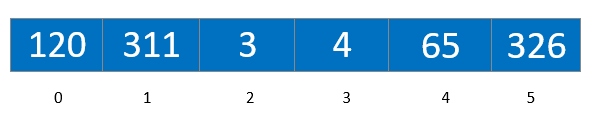Now we copy this array to the original array and perform the above procedure for other passes.

After the execution of each and every pass, our final array will be sorted.

Let’s understand this algorithm from its code.

Code:

## C++ Code

``````#include <iostream>

using namespace std;

void countSort(int arr[], int n, int place) {
int count;
for (int i = 0; i < 10; i++) count[i] = 0;
for (int i = 0; i < n; i++) count[(arr[i] / place) % 10]++; // building the count array
for (int i = 1; i < 10; i++) count[i] = count[i - 1] + count[i]; // modifying the count array

// building the output array
int res[n];
for (int i = n - 1; i >= 0; i--) {
res[count[(arr[i] / place) % 10] - 1] = arr[i];
count[(arr[i] / place) % 10]--;
}
for (int i = 0; i < n; i++) arr[i] = res[i];
}
void radixSort(int arr[], int n) {
// finding the maxElement
int maxElement = arr;
for (int i = 0; i < n; i++) {
maxElement = max(maxElement, arr[i]);
}

// for every digit in the maxElement, running count sort
for (int place = 1; maxElement / place > 0; place *= 10) {
countSort(arr, n, place);
}
for (int i = 0; i < n; i++) cout << arr[i] << " ";
}
int main() {
int arr [] = { 326, 311, 3, 4, 120, 65};
int n = 6;
return 0;
}``````

Output: 3 4 65 120 311 326

The time complexity of radix sort

Space Complexity of radix sort: O(n+k)

## Java Code

``````public class Main {
static void countSort(int arr[], int n, int place) {
int[] count = new int; // since all element are decimal base, so b=10
for (int i = 0; i < 10; i++) count[i] = 0;
for (int i = 0; i < n; i++) count[(arr[i] / place) % 10]++;
for (int i = 1; i < 10; i++) count[i] = count[i - 1] + count[i];

// building the output array
int[] res = new int[n];
for (int i = n - 1; i >= 0; i--) {
res[count[(arr[i] / place) % 10] - 1] = arr[i];
count[(arr[i] / place) % 10]--;
}
for (int i = 0; i < n; i++) arr[i] = res[i];

}
static void radixSort(int arr[], int n) {
// finding the maximum element
int maxElement = arr;
for (int i = 0; i < n; i++) {
maxElement = Math.max(maxElement, arr[i]);
}
// for every digit in the maxElement, running counting sort function
for (int place = 1; maxElement / place > 0; place *= 10) {
countSort(arr, n, place);
}
for (int i = 0; i < n; i++) System.out.print(arr[i] + " ");
}
public static void main(String args[]) {
int arr [] = { 326, 311, 3, 4, 120, 65};
int n = 6;